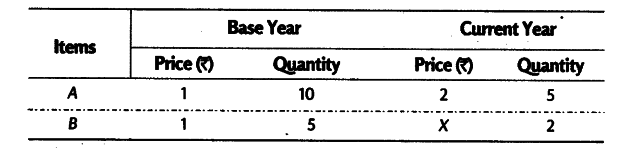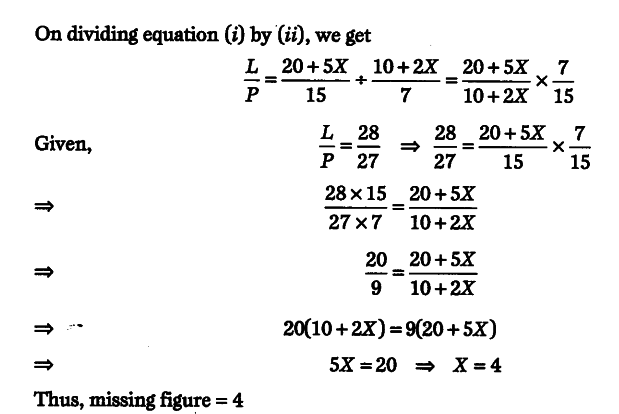# Given the following data, Find X, if the ratio between Laspeyre's and Paasche's index number is 28.27

Given the following dataFind X, if the ratio between Laspeyre’s and Paasche’s index number is 28.27